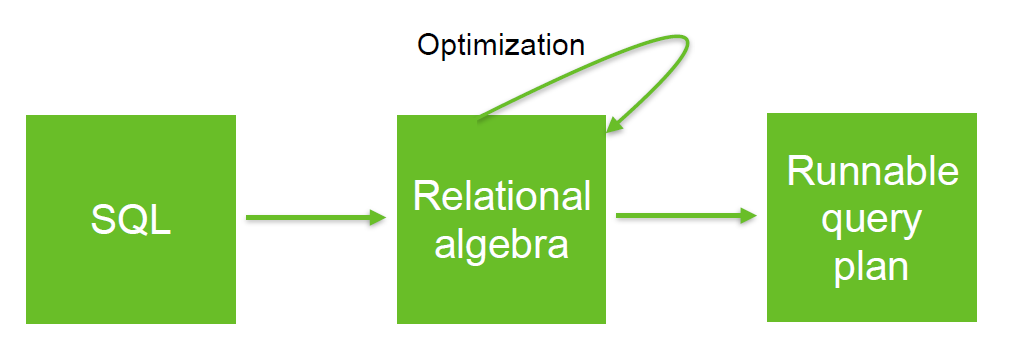# Relational Algebra - Division Operator

The division relational operator permits to find values in an attribute of R that have all values of S in the attribute of the same name

There is no SQL equivalent.

## Symbol

$$\LARGE R \div S \\ or\\ \LARGE R / S$$

## Formula

The division of R by S that return all values of the attributes R.t that have a row with all value in S.s can be expressed as: $$\LARGE R / S \equiv \{\; \left<\vec t\right> \;|\; \forall \left<\vec s\right> \in S, \left< \vec t \vec s \right> \in R \;\}$$ where:

• $\forall$ means for all
• $\vec t \vec s$ are vector

## Documentation / Reference

Discover MoreRelational Algebra - Expression and Operators

Relational algebra is based upon the fact that you can pass tabular data through a set of data operators (select, filter, join, sort, union, etc.) in a algebraic structure. It means that: the output...